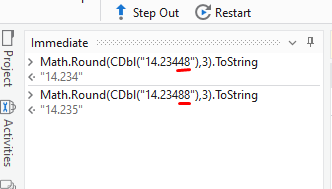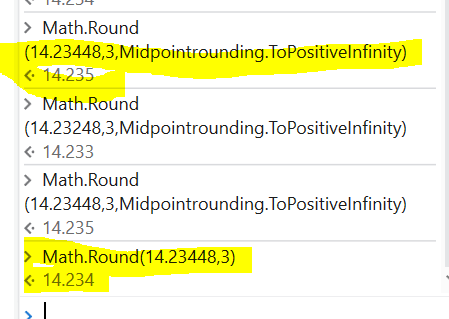# Round off not giving the expected result

Hi All,
I would like to round off any number to 3 decimal places . I tried below methods but not giving the expected results,
sample = 14.23448
Method1 :
CDbl(sampl).ToString(“#.###”) gives 14.234 but the expected result is 14.235 since the last digit is 8.While converting, 4th decimal should be 5 and in turn the 3rd decimal be 5
Method 2:Same Problem
Math.Round(CDbl(sampl),3).ToString = 14.234 but the expected result is 14.235
Method 3: Same problem
CDbl(sampl).ToString(“#.000”) = 14.234 but the expected result is 14.235

@raja.raviraj

I think you need to perform round of for 4 th decimal place first and again you need to do same for 3 rd decimal position.

If you want to round off 14.23448 to 3 decimal places

• Go to the decimal point: 14.23448

• Count along the first 3 numbers to the right: 14.23448

• Look at the next number: 14.23448

• It is less than 5, so leave the 3rd number as it is and drop all the numbers after it: 14.234

If you want to round off 14.23488 to 3 decimal places

• Go to the decimal point: 14.23488

• Count along the first 3 numbers to the right: 14.23488

• Look at the next number: 14.23488

• It is greater than or equal to 5, so increase the 3rd number by 1 and drop all the numbers after it: 14.235.

After the Decimal point it should be greater than 5, If so it will return `14.235`. If not it will return `14.234`

You can see the differ in the imageRegards
Gokul

@raja.raviraj

Try like this

`Math.Round("number",3,Midpointrounding.AwayFromZero)`

Or

`Math.Round("number",3,Midpointrounding.ToPositiveInfinity)`

Cheers

1 Like

You can use the `Math.Round()` method to achieve the desired result. However, you need to use the overload that takes the `MidpointRounding` parameter to specify how to handle rounding of numbers that are exactly halfway between two possible rounded values.

In your case, you can use `MidpointRounding.AwayFromZero` to ensure that rounding is always away from zero, so that numbers that are exactly halfway between two possible rounded values are rounded up.

Here’s an example:

``````Dim sample As Double = 14.23448
Dim roundedValue As Double = Math.Round(sample, 3, MidpointRounding.AwayFromZero)
``````

The `roundedValue` variable will contain the value 14.235, which is the expected result.

Thanks. This didn’t work.Faced the same issue

@raja.raviraj`Math.Round(14.23448,3,Midpointrounding.ToPositiveInfinity)`